Making audio from scratch is very cool and surprisingly easy!

Let’s step back a level and ask: what is sound? A physicist would tell you it’s repeated pressure variation in the air – compression and rarefaction – which passes through the medium. If you were to pick a point, and plot the pressure at that point over time –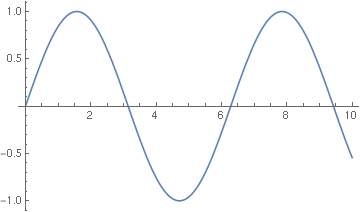The problem with this is its continuity. Mathematically and physically, this is a purely continuous behaviour. Computers are really, really bad at dealing with continuity; it’s much easier to sample at some rate. Here, I take a sample every 1 unit of time.That’s not a particularly accurate representation of the wave. Higher sample rates are more faithful; here, I sample every 0.1 units of time.Sampling sound becomes vaguely recognisable at a rate of thousands of samples per second. (Given we’re talking about a frequency, let’s use the correct unit: hertz). 8000 Hz is roughly what a telephone call sounds like; CDs sample at 44,100 Hz, DVD video at 48,000 Hz, and most content online uses one of these two common rates.

(Those rates are particularly interesting, because of a strange quirk called the Nyquist frequency: we can only capture waves at half the frequency we sample at; above that, the waves tend to alias and effectively disappear. The human hearing bottoms out somewhere around 20 kHz, so it makes no sense to capture at a higher rate, because the sound reproduced would be practically inaudible. Some hi-fi enthusiasts disagree.)

The rate at which we sample the signal is not the only important part: we also need some resolution. Let’s say we have one sampled bit every second – that is, every time we sample our signal, we only record one bit of information about the signal; here, a signal ≥ 0 gives 1, and a signal < 0 gives 0.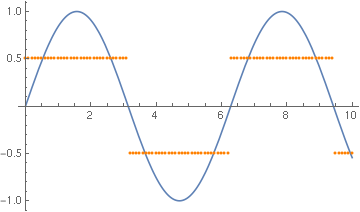The samples here are almost totally unrecognisable as the original signal! Clearly, we need more bits; how about two?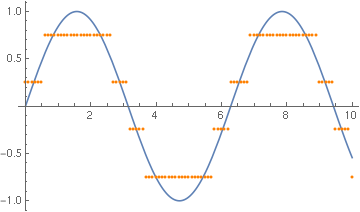Clearly, we need more bits; how about four?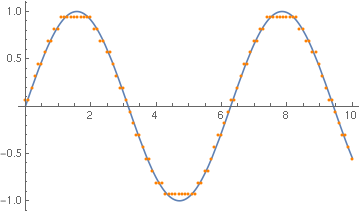Clearly, we need more bits; how about eight?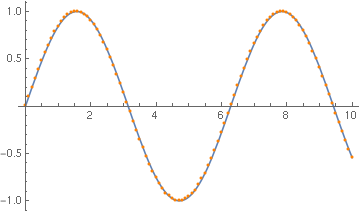By capturing more information at each sample, we can more accurately represent the signal. Common sample resolutions for audio are 8-bit, 16-bit, and 24-bit.

These samples can be collected with an analogue-digital converter, or ADC – a device that turns an electrical signal into a bit-stream. The opposite device, a digital-analogue converter, or DAC, also exists; it turns a bitstream into a signal, often by wiggling its output level in a certain way. (I would consider both of these devices to be sufficiently advanced technology that we can treat as a magical black box; I’m not an electrical engineer.)

We now have all the information we need: we have a stream of samples at a particular rate, each sample carrying an amount of information, and given that stream, rate, and resolution, we can reproduce that signal.

For our purposes, we’ll run at 8000 Hz, at 8 bits. On my (Linux) computer, I can take such a bitstream and play it back directly, using a command called `play` (a part of SoX, the Sound eXchange tools).

We need to tell `play` about our input format, which means it needs several command-line arguments: `-r|--rate` sets the sample rate, `-b|--bits` sets the sample resolution, `-t|--type` sets the sample stream type, `-e|--encoding` sets the encoding of each sample, and `-L|--endian little` specifies that samples are little-endian. (Most music file formats pack all this information into their file header, so you don’t have to specify how to set up a DAC every time you want to listen.)

To make sure you can hear something, try piping the “random” pseudo-file into `play`. `/dev/urandom` is a device file which generates as much random data as you like –

``````\$ cat /dev/urandom | play -r 8000 -b 8 -t raw -e unsigned -L -
``````

This should produce “white noise”: a totally random spread of signal, over the entire spectrum.

We can produce these samples ourselves, by plugging our own programs into this pipeline.

Here’s a simple program that generates a continuous stream of samples. Each sample is eight bits – one byte – long, and we keep it in `i` as we go. We rely on `i` wrapping around: this is a fairly common behaviour, but strictly is undefined, so `dcc` won’t like this much.

``````#include <stdint.h> // for uint8_t
#include <stdio.h>

int
main (void)
{
uint8_t i = 0;
while (1) {
putchar (i);
i++;
}
}
``````

If you run this, and dump it to your terminal, you’ll get a screenful of garbage, and probably also some random bells. The terminal is attempting to make sense of this data as ASCII, which works roughly half the time. But if I pipe this into `play`, I get a weird buzzing noise. Hooray!

What’s actually happening here is we’ve produced a sawtooth wave. It wraps around every 256 samples, so roughly at 31.25 Hz.A simple FFT application on my phone suggests this is a very noisy signal indeed – we’ll come back to why that is shortly.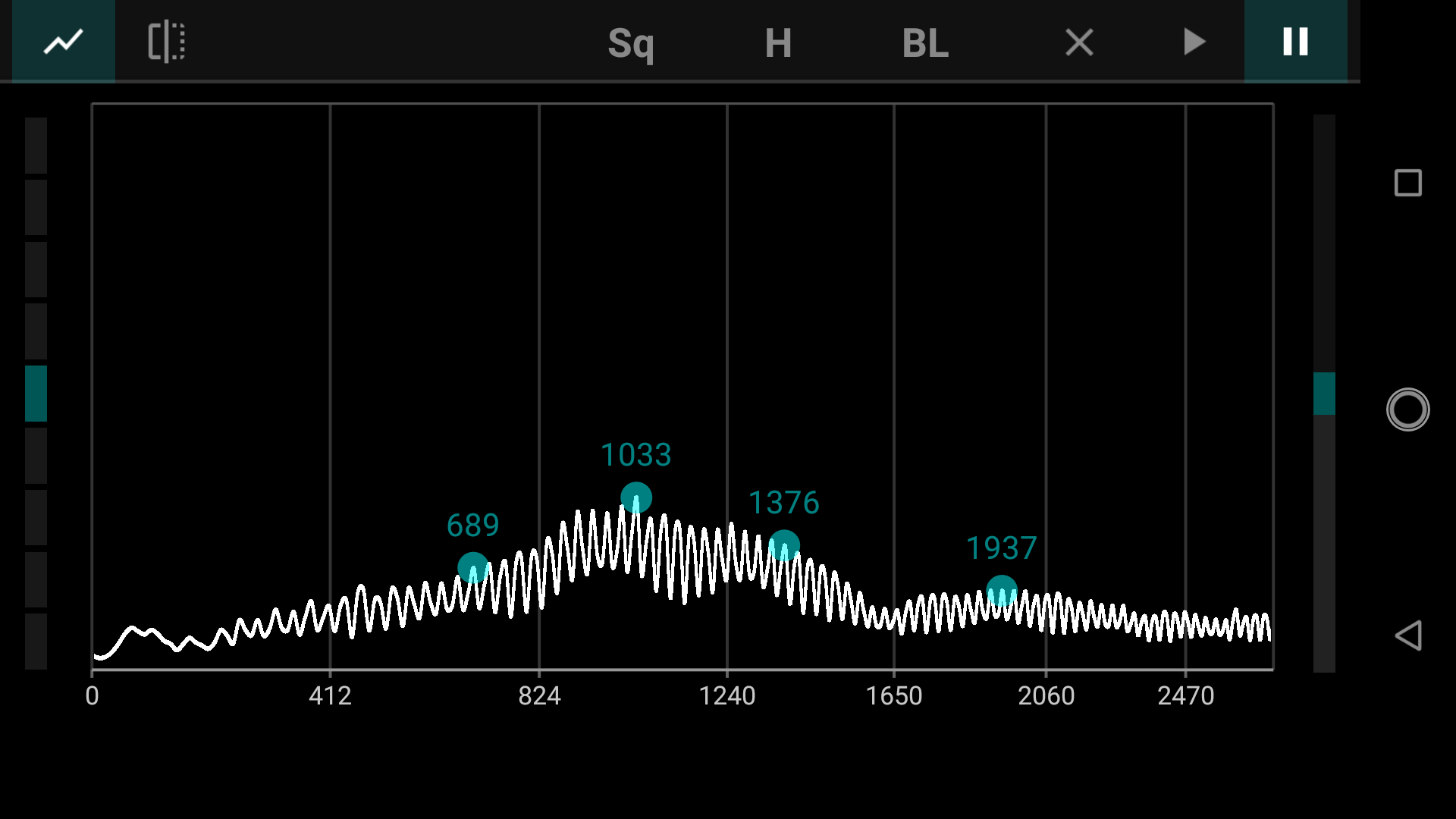A Fourier transform is mathemagical device for turning a time-series signal into a frequency-series signal. It decomposes a signal over time into a sum of trigonometric functions, which (very closely) approximate the original value. Most signal processing relies on transforms like this. In particular, the overtones are very obvious.

Sawtooth waves don’t decompose particularly nicely in this way. Here’s an example from the Mathematica documentation, showing what’s going on here.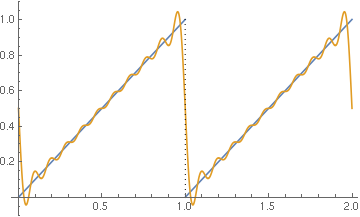(Thought: how might you produce a nicer tone? You may want to think about functions in `<math.h>`.)

Here’s a simple function that goes further: it takes a frequency in hertz and a duration, and plays a note. We know that going up one step every sample produces a sawtooth wave at 31.25 Hz, so by dividing that out, we can produce arbitrary frequencies!

``````static void
play_note (double hz, double sec)
{
int i = 0;
double out = 0.0;
double step = (U8_RANGE * hz) / SAMPLE_RATE;

while (i < SAMPLE_RATE * sec) {
putchar ((uint8_t) out);
i++; out += step;
}
}
``````

In this case, we use a floating-point value to make the function simpler. This is ‘good enough’ for our purposes: we’re unlikely to hold onto the tone for a particularly long time, and we cast it down to the `uint8_t` type anyway.
``````    play_note (440.000, 1.0);
And, of course, pipe this into `play`, I get a hopefully recognisable note!
This still sounds particularly unpleasant, but given an array of frequency/duration pairs, if we call `play_note` on each, we could wind up with a possibly recognisable song. (If you’ve forgotten what it is, that’s a painstakingly hand-transcribed version of Wintergatan’s Marble Machine.) (download marbles.c)